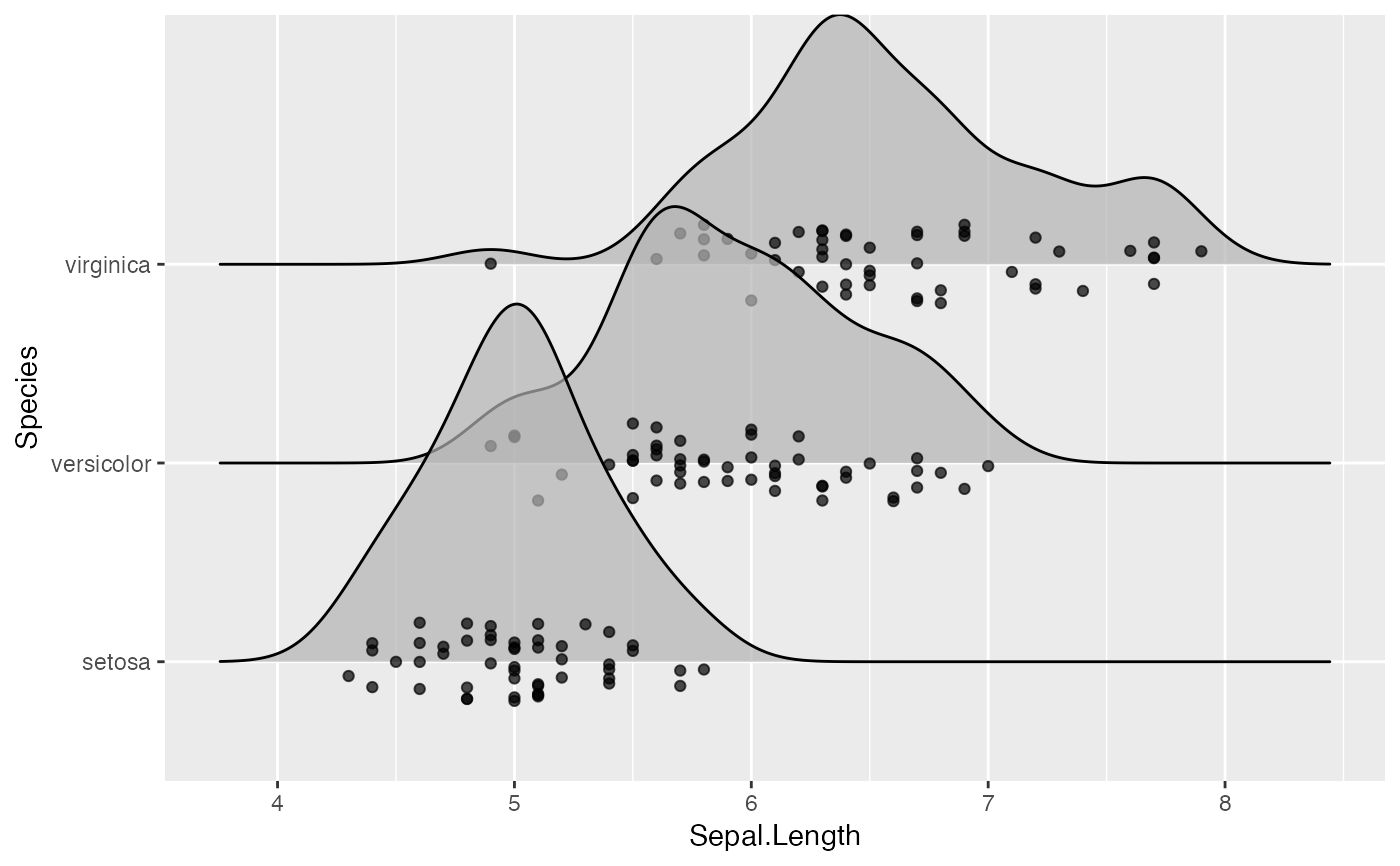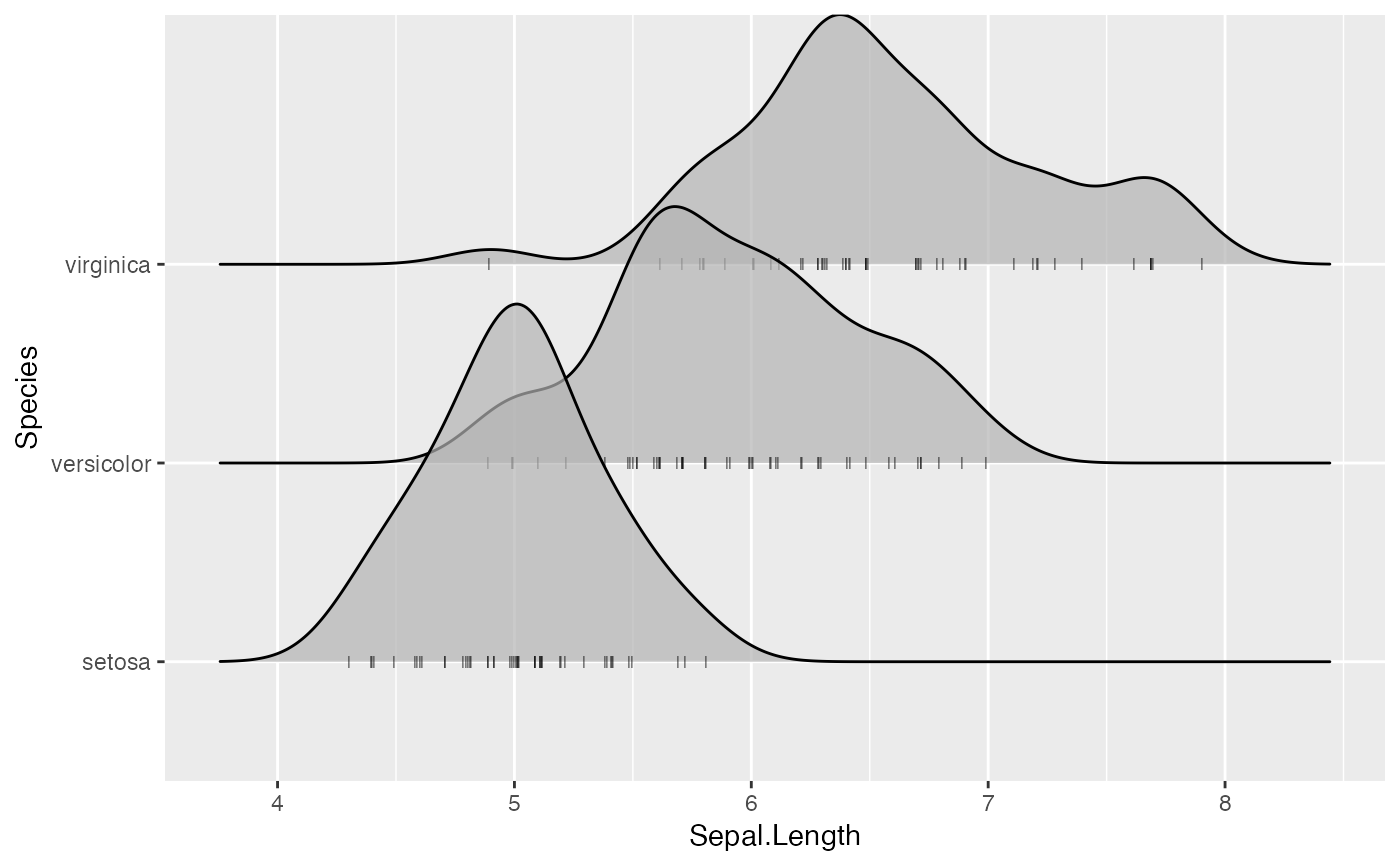This is a position adjustment specifically for geom_density_ridges() and related geoms. It only jitters the points drawn by these geoms, if any. If no points are present, the plot remains unchanged. The effect is similar to position_jitter(): points are randomly shifted up and down and/or left and right.

position_points_jitter(
width = 0,
height = 0.2,
yoffset = 0,
seed = NULL
)

## Arguments

width

Width for horizontal jittering. By default set to 0.

height

Height for vertical jittering, applied in both directions (up and down). By default 0.2.

yoffset

Vertical offset applied in addition to jittering.

If TRUE, adjusts vertical lines (as are drawn for quantile lines, for example) to align with the point cloud.

seed

Random seed. If set to NULL, the current random number generator is used. If set to NA, a new random random seed is generated. If set to a number, this number is used as seed for jittering only.

Other position adjustments for ridgeline plots: position_points_sina, position_raincloud

## Examples

library(ggplot2)

# default jittered points
ggplot(iris, aes(x = Sepal.Length, y = Species)) +
geom_density_ridges(jittered_points = TRUE, position = "points_jitter", alpha = 0.7)
#> Picking joint bandwidth of 0.181# simulating a rug
ggplot(iris, aes(x = Sepal.Length, y = Species)) +
geom_density_ridges(jittered_points = TRUE, point_shape = '|', alpha = 0.7, point_size = 2,
position = position_points_jitter(width = 0.02, height = 0))
#> Picking joint bandwidth of 0.181